Tailwater
The Tailwater methods (available in the Tailwater category of the User Selectable Methods) calculate the Tailwater Elevation of a Level Power Reservoir. The Tailwater Elevation represents the water surface elevation immediately downstream of the Power Plant. This parameter is required to calculate the Operating Head of the Power Reservoir which is used to calculate either the Energy or Turbine Release of that Reservoir. The Tailwater methods are dependent upon the Reservoir being a Level Power Reservoir and a valid Power method (available in the Power category of the User Selectable Methods) being selected. These methods require a valid Outflow to perform their calculations.None
This is the default method in the Tailwater category. This method performs no calculations.
There are no slots specific to this method.Linked or Input
The Linked or Input method allows the user to either input values for the Tailwater Elevation or link the Tailwater Base Value to a slot (Pool Elevation or Backwater Elevation) on a downstream Reservoir. If the Tailwater Base Value is linked, the Tailwater Elevation cannot be input by the user. If the Tailwater Base Value is not linked, the Tailwater Elevation must be input by the user. The Tailwater Elevation is determined by following procedure if it is not input (that is, the Tailwater Base Value is linked).
Slots Specific to This Method
Tailwater Base Value
Type: Series
Units: LENGTH
Description: elevation of tailwater or base elevation used to compute elevation of tailwater
Information:
I/O: Optional; may be input or set by a link.
Method Details
The Linked or Input user method does not perform any calculations, specifically. There are however many logical evaluations performed in this method. The Linked or Input method is discussed below.
If a value for the Tailwater Base Value has been calculated by another user method or propagated through a link, the temporary value for the Tailwater Base Value (TWBaseValueTemp) is set equal to the calculated or linked Tailwater Base Value.
If the Tailwater Base Value is linked to another slot, the following process is performed.
1. If the Tailwater Elevation is input, a RiverWare error is flagged and the run terminates.
2. If TWBaseValueTemp is known, the Tailwater Elevation is set equal to the TWBaseValueTemp.
3. If TWBaseValueTemp is not known, but the previous timestep’s Tailwater Elevation is known, the Tailwater Elevation is set equal to the previous timestep’s Tailwater Elevation.
4. If neither the TWBaseValueTemp nor the previous timestep’s Tailwater Elevation is known and the previous timestep was the initial timestep of the simulation, a RiverWare error is flagged and the simulation run terminates.
5. If neither the TWBaseValueTemp nor the previous timestep’s Tailwater Elevation is known and the previous timestep was not the initial timestep, the method is exited and waits for more information.
If the Tailwater Base Value is not linked to another slot, Tailwater Elevation must be input. If the Tailwater Elevation is not input, a RiverWare error is flagged and the simulation run terminates.Base Value Only
The Base Value Only method is similar to the Linked or Input method. If this method is selected, the user must either input values directly into the Tailwater Base Value slot or link the Tailwater Base Value slot to either the Pool Elevation slot or the Backwater Elevation slot of the downstream Reservoir. Either the initial Tailwater Elevation or the initial Tailwater Base Value must be input by the user for this method to execute successfully. The Tailwater Elevation for any timestep is computed as the average Tailwater Base Value over the timestep.
Slots Specific to This Method
Tailwater Base Value
Type: Series
Units: LENGTH
Description: elevation of tailwater or base elevation of tailwater is used to compute the tailwater elevation
Information:
I/O: Optional; may be input or linked.
Method Details
This method is based on logic similar to that of the Linked or Input method. The Base Value Only user method performs many logical evaluations to set the Tailwater Elevation. This method is described below.
The temporary Tailwater Base Value (TWBaseValueTemp) is set equal to the Tailwater Base Value if the Tailwater Base Value was calculated by another user method or set via a link.
• If the Tailwater Base Value is linked to another slot, the following process is performed.
1. If the previous timestep’s Tailwater Base Value is not known, and both the previous timestep’s Tailwater Elevation and TWBaseValueTemp are known, the Tailwater Elevation is calculated.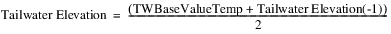where Tailwater Elevation (-1) is the previous timestep’s Tailwater Elevation.
2. If neither the previous timestep’s Tailwater Base Value nor the TWBaseValueTemp is known but the previous timestep’s Tailwater Elevation is known, the current timestep is set equal to the previous timestep’s Tailwater Elevation.
3. If neither the previous timestep’s Tailwater Base Value nor the previous timestep’s Tailwater Elevation is known and the previous timestep is the initial timestep, a RiverWare error is posted and the simulation run terminates.
4. If neither the previous timestep’s Tailwater Base Value nor the previous timestep’s Tailwater Elevation is known and the previous timestep is not the initial timestep, more information must be known. The method is exited and waits for more information to be known.
5. If both the previous timestep’s Tailwater Base Value and the TWBaseValueTemp are known, the Tailwater Elevation is calculated.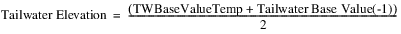6. If the previous timestep’s Tailwater Base Value is known but TWBaseValueTemp is not known, Tailwater Elevation is set equal to the previous timestep’s Tailwater Base Value.
• If the Tailwater Base Value is not linked, the following process is performed.
1. If the Tailwater Elevation is not known and the TWBaseValueTemp is known, the Tailwater Elevation is set equal to the TWBaseValueTemp.
2. If neither the Tailwater Elevation nor the TWBaseValueTemp are known, a RiverWare error is flagged and the simulation run terminates.Base Value Plus Lookup Table
The Base Value Plus Lookup Table method computes the Tailwater Elevation. This is done by adding the average Tailwater Base Value (over the timestep) to a function of Outflow determined by the Tailwater Table. The Outflow value used to find the corresponding Tailwater value on the Tailwater Table is either the value of the local variable Flow or the value of the Outflow slot. The Tailwater Base Value may be input by the user or linked to either the Pool Elevation slot or the Backwater Elevation slot of a downstream Reservoir. If the Tailwater Base Value is neither input nor linked, it is assumed to be zero.
Slots Specific to This Method
Tailwater Base Value
Type: Series
Units: LENGTH
Description: base elevation of the tailwater
Information:
I/O: Optional; can be input or linked.
Tailwater Table
Type: Table
Units: FLOW vs LENGTH
Description: reservoir outflow vs either the tailwater elevation or the tailwater elevation increment
Information: If the Tailwater Base Value is non-zero, the Tailwater Table gives values of incremental increase in Tailwater Elevation over the Base value. Otherwise, the table gives the Tailwater Elevation values.
I/O: Required input
Method Details
This method is based on logic similar to that of the Linked or Input method. The Base Value Plus Lookup Table user method performs many logical evaluations to set the Tailwater Elevation. This method is described below.
The temporary Tailwater Base Value (TWBaseValueTemp) is set equal to the Tailwater Base Value if the Tailwater Base Value was calculated by another user method or set via a link.
If the Local Info variable Flow is known, the local variable, tempflow, is set equal to Flow. If the Local Info variable Flow is not known, but the Outflow is known, tempflow is set equal to Outflow.
If the tempflow value is known, the following steps are taken to determine the Tailwater Elevation.
If the Tailwater Base Value is linked (and tempflow is known), the following process is performed.
1. If both TWBaseValueTemp and the previous timestep’s Tailwater Base Value are known, TWTemp (a local variable) is obtained from a table interpolation performed on the Tailwater Table using tempflow. The Tailwater Elevation is then calculated.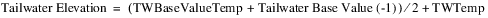2. If TWBaseValueTemp is known but the previous timestep’s Tailwater Base Value is known, but the previous timestep’s Tailwater Basic Value is not known. TWTemp is obtained from a table interpolation performed on the Tailwater Table using tempflow. The Tailwater Elevation is then calculated.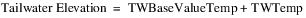3. If TWBaseValueTemp is not known but the previous timestep’s Tailwater Base Value is known, TWTemp is obtained from a table interpolation performed on the Tailwater Table using tempflow. The Tailwater Elevation is then calculated.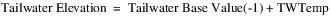4. If neither TWBaseValueTemp nor the previous timestep’s Tailwater Base Value are known but the previous timestep’s Tailwater Elevation is known, the current timestep’s Tailwater Elevation is set equal to the previous timestep’s Tailwater Elevation.
5. If neither TWBaseValueTemp nor the previous timestep’s Tailwater Base Value is known and the previous timestep is the initial timestep of the run, a RiverWare error is posted and the run terminates.
6. If neither the TWBaseValueTemp nor the previous timestep’s Tailwater Base Value is known and the previous timestep is not the initial timestep of the run, the method is exited and waits for more information.
If the Tailwater Base Value is not linked (and tempflow is known), the following process is performed.
1. If TWBaseValueTemp is known, TWTemp is obtained from a table interpolation performed on the Tailwater Table using tempflow. The Tailwater Elevation is then calculated.2. If TWBaseValueTemp is not known, the Tailwater Elevation is obtained from a table interpolation performed on the Tailwater Table using tempflow.
The only case where tempflow is not known is when Outflow is not known. This scenario only occurs at the first timestep in one of the three given Energy dispatch methods. The following steps are performed to determine the Tailwater Elevation if tempflow is not known.
If the Tailwater Base Value is linked (and tempflow is not known), the following process is performed.
1. If TWBaseValueTemp and the previous timestep’s Tailwater Elevation are known, the Tailwater Elevation is calculated.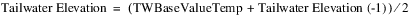2. If both TWBaseValueTemp and the previous timestep’s Tailwater Base Value are known but the previous timestep’s Tailwater Elevation is not known, Tailwater Elevation is calculated.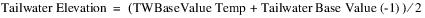3. If TWBaseValueTemp is known, but neither the previous timestep’s Tailwater Elevation nor the previous timestep’s Tailwater Base Value are known, Tailwater Elevation is set equal to TWBaseValueTemp.
4. If TWBaseValueTemp is not known but the previous timestep’s Tailwater Elevation is known, the current timestep’s Tailwater Elevation is set equal to the previous timestep’s Tailwater Elevation.
5. If neither TWBaseValueTemp nor the previous timestep’s Tailwater Elevation are known, but the previous timestep’s Tailwater Base Value is known, Tailwater Elevation is set equal to the previous timestep’s Tailwater BaseValue.
6. If the previous timestep is the initial timestep of the run, and none of the following are known: TWBaseValueTemp, the previous timestep’s Tailwater Base Value, and the previous timestep’s Tailwater Elevation, a RiverWare error is posted and the simulation run terminates.
7. If the previous timestep is NOT the initial timestep of the run, and none of the following are known: TWBaseValueTemp, the previous timestep’s Tailwater Base Value, and the previous timestep’s Tailwater Elevation, the method is exited and waits for more information to execute.
If the Tailwater Base Value is not linked (and tempflow is NOT known), the following process is performed.
1. If the Tailwater Elevation is known, the method is exited because no calculations need to be performed.
2. If the Tailwater Elevation is NOT known, and TWBaseValueTemp is NOT equal to zero, Tailwater Elevation is set equal to TWBaseValueTemp.
3. If the Tailwater Elevation is NOT known and either the temporary Tailwater Base Value is NOT known or equal to zero or both, Tailwater Elevation is set equal to the elevation corresponding to zero on the Tailwater Table.
Related Terms
Following are definitions of some terms used in the these equations.
• TWBaseValueTemp—Temporary value for the Tailwater Base Value. This value may be determined from another method or propagated across a link.
• tempflow—Local variable used as the outflow from the Reservoir.
• TWTemp—Local variable used to represent the incremental increase in the Tailwater Elevation over the Tailwater Base Value.
• Tailwater Elevation (-1)—Tailwater Elevation at the previous timestep.
• Tailwater Base Value (-1)— Tailwater Base Value at the previous timestep.Stage Flow Lookup Table
The Stage Flow Lookup Table method is similar to the other methods for determining Tailwater Elevation. The Tailwater Elevation is obtained from a three-dimensional table relating Outflow, Downstream Stage, and the corresponding Tailwater Elevation for most cases. The data in this table must be input by the user. The value for Downstream Stage that is used in this method is the larger of the Tailwater Reference Elevation or the Tailwater Base Value if the Tailwater Base Value is linked to a downstream elevation. If the Tailwater Base Value is not linked, the temporary Tailwater Base Value is used as the Downstream Stage. An average value for the Tailwater Elevation over the timestep is used whenever possible.
Slots Specific to This Method
Stage Flow Tailwater Table
Type: Table
Units: FLOW vs LENGTH vs LENGTH
Description: Reservoir Outflow vs Downstream Elevation (Tailwater Base Value) vs Tailwater Elevation
Information: Data must be entered into the table in increasing blocks of the same Outflow value for the three-dimensional table interpolator to work correctly. For every block of same Outflows in column 1, Stages should be listed in increasing order in column 2, and the corresponding Tailwater Elevations in column 3.

Outflow
Downstream Stage
TW Elevation
100
500
510
100
550
560
100
600
610
200
500
520
200
550
570
200
600
620
300
500
530
300
550
580
300
600
630
I/O: Required input
Tailwater Base Value
Type: Series
Units: LENGTH
Description: base elevation of the tailwater
Information:
I/O: Optional; can be input or set by a link.
Tailwater Reference Elevation
Type: Table
Units: LENGTH
Description: lowest Reservoir discharge Elevation when there are no backwater effects from a downstream pool (reservoir)
Information: If this slot has input data, the greater of the Tailwater Reference Elevation or the linked lower reservoir’s Pool or Backwater Elevation is used to calculate the Tailwater Base Value. If the Tailwater Base Value is linked to a downstream elevation, this value must be specified by the user. If the Tailwater Base Value is not linked, this value is not used, even if specified.
I/O: Required input
Method Details
The Stage Flow Lookup Table user method performs many logical tests to determine the Tailwater Elevation. This method is described below.
1. The first step in this method is to check and see if the Tailwater Reference Elevation is known. If the Tailwater Reference Elevation is not known, a RiverWare error is posted and the run terminates.
2. The temporary Tailwater Base Value (TWBaseValueTemp) is set equal to the Tailwater Base Value if the Tailwater Base Value was calculated by another user method or set via a link.
3. If the local variable Flow is known, the local variable tempflow is set equal to Flow If the local variable Flow is not known, but the Outflow is known, if the Local Info variable Flow is not known but Outflow is known, tempflow is set equal to Outflow.
4. If the tempflow value is known, the following steps are taken to determine the Tailwater Elevation.
If the Tailwater Base Value is linked (and tempflow is known), the following process is performed.
1. If both TWBaseValueTemp and the previous timestep’s Tailwater Base Value are known, downstreamStage is computed as the average of the previous timestep’s Tailwater Base Value and either the Tail Water Reference Elevation or TWBaseValueTemp (whichever is greater). A table interpolation is performed to determine the Tailwater Elevation using the downstreamStage, tempflow, and the Stage Flow Tailwater Table.
2. If TWBaseValueTemp is known but the previous timestep’s Tailwater Base Value is NOT known, downstreamStage is set equal to either the Tail Water Reference Elevation or TWBaseValueTemp (whichever is greater). A table interpolation is performed to determine the Tailwater Elevation using the downstreamStage, tempflow, and the Stage Flow Tailwater Table.
3. If the previous timestep’s Tailwater Base Value is known but TWBaseValueTemp is NOT known, downstreamStage is set equal to the previous timestep’s Tailwater Base Value. A table interpolation is performed to determine the Tailwater Elevation using the downstreamStage, tempflow, and the Stage Flow Tailwater Table.
4. If neither TWBaseValueTemp nor the previous timestep’s Tailwater Base Value are known, but the previous timestep’s Tailwater Elevation is known, the current timestep’s Tailwater Elevation is set equal to the previous timestep’s Tailwater Elevation.
5. If the previous timestep was the initial timestep and none of the following are known: TWBaseValueTemp, the previous timestep’s Tailwater Base Value, and the previous timestep’s Tailwater Elevation, a RiverWare error is posted and the run terminates.
6. If the previous timestep was NOT the initial timestep, and none of the following are known: the temporary TWBaseValueTemp, the previous timestep’s Tailwater Base Value, and the previous timestep’s Tailwater Elevation, the method is exited and waits for more information.
If the Tailwater Base Value is not linked (and tempflow is known), the following process is performed.
1. If TWBaseValueTemp is known, downstreamStage is set equal to TWBaseValueTemp. A table interpolation is performed to determine the Tailwater Elevation using the downstreamStage, tempflow, and the Stage Flow Tailwater Table.
2. If TWBaseValueTemp is not known, a RiverWare error is posted and the run terminates.
The only case where tempflow is not known is when Outflow is not known. This scenario only occurs at the first timestep in one of the three given Energy dispatch methods. The following steps are performed to determine the Tailwater Elevation if tempflow is not known.
If the Tailwater Base Value is linked (and tempflow is not known), the following process is performed.
1. If both TWBaseValueTemp and the previous timestep’s Tailwater Elevation are known, the Tailwater Elevation is calculated.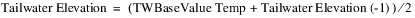2. If both TWBaseValueTemp and the previous timestep’s Tailwater Base Value are known but the previous timestep’s Tailwater Elevation is not known, Tailwater Elevation is computed.3. If TWBaseValueTemp is known but neither the previous timestep’s Tailwater Elevation nor the previous timestep’s Tailwater Base Value are known, Tailwater Elevation is set equal to TWBaseValueTemp.
4. If TWBaseValueTemp is not known but the previous timestep’s Tailwater Elevation is known, the current timestep’s Tailwater Elevation is set equal to the previous timestep’s Tailwater Elevation.
5. If neither TWBaseValueTemp nor the previous timestep’s Tailwater Elevation is known, but the previous timestep’s Tailwater Base Value is known, Tailwater Elevation is set equal to the previous timestep’s Tailwater Base Value.
6. If the previous timestep is the initial timestep of the run, and none of the following are known: TWBaseValueTemp, the previous timestep’s Tailwater Base Value, and the previous timestep’s Tailwater Elevation, a RiverWare error is posted, and the run terminates.
7. If the previous timestep is NOT the initial timestep of the run and none of the following are known: TWBaseValueTemp, the previous timestep’s Tailwater Base Value, and the previous timestep’s Tailwater Elevation, the method is exited and waits for more information.
If the Tailwater Base Value is not linked (and tempflow is not known) the following process is performed.
1. If the Tailwater Elevation is known, the method is exited because no calculations need to be performed.
2. If the Tailwater Elevation is NOT known and the TWBaseValueTemp is NOT equal to zero, Tailwater Elevation is set equal to TWBaseValueTemp.
3. If the Tailwater Elevation is NOT known and either the temporary Tailwater Base Value is NOT known or equal to zero or both, Tailwater Elevation is set equal to the elevation corresponding to zero on the Tailwater Table.
Related Terms
Following are definitions of some terms used in these equations.
• TWBaseValueTemp—Temporary value for the Tailwater Base Value. This value may be determined from another method or propagated across a link.
• tempflow—Local variable used as the outflow from the Reservoir.
• downstreamStage—Local variable used to hold the value of the downstream stage elevation.
• Tailwater Elevation (-1)—Tailwater Elevation at the previous timestep.
• Tailwater Base Value (-1)—Tailwater Base Value at the previous timestep.Compare to Avg Base Value
The Compare to Avg Base Value method uses similar methodology as the Base Value Plus Lookup Table and Stage Flow Lookup Table methods. The only difference between this method and the others is that a new local variable, TWCompare, is used. The value for TWCompare is compared with a table lookup value for the Tailwater Elevation. The larger of the two values is used as the Tailwater Elevation. An average Tailwater Elevation over the timestep is calculated whenever possible.
Slots Specific to This Method
Tailwater Base Value
Type: Series
Units: LENGTH
Description: base elevation of the tailwater
Information:
I/O: Optional; can be input of set by a link.
Tailwater Table
Type: Table
Units: FLOW vs LENGTH
Description: Reservoir Outflow vs either the Tailwater Elevation or the tailwater elevation increment
Information: If the Tailwater Base Value is non-zero, the Tailwater Table holds values of incremental increase in Tailwater Elevation over the Base value. Otherwise, the table holds the Tailwater Elevation values.
I/O: Required input
Method Details
The Compare to Avg Base Value user method performs many logical evaluations to determine the Tailwater Elevation. This method is described below.
1. The temporary Tailwater Base Value (TWBaseValueTemp) is set equal to the Tailwater Base Value is the Tailwater was calculated by another user method or set via a link. For the Tailwater Compare Method, the Tailwater Base Value must be linked to another object. Otherwise there would be no reason to compare values. If the Tailwater Base Value is not linked, a RiverWare error is posted and the simulation run terminates.
2. If the Local Info variable Flow is known, the local variable tempflow is set equal to Flow.
3. If the local variable Flow is not known, but Outflow is known, tempflow is set equal to Outflow.
If the tempflow value is known, the following steps are taken to determine the Tailwater Elevation.
1. If both TWBaseValueTemp and the previous timestep’s Tailwater Base Value are known,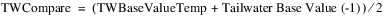2. If TWBaseValueTemp is known but the previous timestep’s Tailwater Base Value is NOT known, TWCompare is set equal to TWBaseValueTemp.
3. If TWBaseValueTemp is not known but the previous timestep’s Tailwater Base Value is known, TWCompare is set equal to the previous timestep’s Tailwater Base Value.
4. If neither TWBaseValueTemp nor the previous timestep’s Tailwater Base Value are known and the previous timestep is the initial timestep, a RiverWare error is posted and the run terminates.
5. If neither TWBaseValueTemp nor the previous timestep’s Tailwater Base Value are known and the previous timestep is NOT the initial timestep, the method is exited and waits for more information.
Once the TWCompare value has been determined, a table interpolation is performed using the Tailwater Table to obtain the tailwater elevation corresponding to the tempflow value. The tailwater elevation value obtained from this interpolation is then compared to TWCompare. The largest of the two values is used to set the Tailwater Elevation.
The only case where tempflow is not known is when Outflow is not known. This scenario only occurs at the first timestep in one of the three given Energy dispatch methods. The following steps are performed to determine the Tailwater Elevation if tempflow is not known.
1. If both TWBaseValueTemp and the previous timestep’s Tailwater Elevation are known,2. If both TWBaseValueTemp and the previous timestep’s Tailwater Base Value are known but the previous timestep’s Tailwater Elevation is not known,3. If TWBaseValueTemp is linked and the temporary Tailwater Base Value is known but neither the previous timestep’s Tailwater Elevation nor the previous timestep’s Tailwater Base Value are known, the Tailwater Elevation is set equal to TWBaseValueTemp.
4. If TWBaseValueTemp is not known but the previous timestep’s Tailwater Elevation is known, the current timestep’s Tailwater Elevation is set equal to the previous timestep’s Tailwater Elevation.
5. If neither TWBaseValueTemp nor the previous timestep’s Tailwater Elevation are known, but the previous timestep’s Tailwater Base Value is known, the current timestep’s Tailwater Elevation is set equal to the previous timestep’s Tailwater Base Value.
6. If the previous timestep is the initial timestep of the run, and none of the following are known: TWBaseValueTemp, the previous timestep’s Tailwater Base Value, and the previous timestep’s Tailwater Elevation, a RiverWare error is posted and the simulation terminates.
7. If the previous timestep is NOT the initial timestep of the run and none of the following are known: TWBaseValueTemp, the previous timestep’s Tailwater Base Value, and the previous timestep’s Tailwater Elevation, the method is exited and waits for more information.
Related Terms
Following are definitions of some terms used in these equations.
• TWBaseValueTemp—Temporary value for the Tailwater Base Value. This value may be determined from another method or propagated across a link.
• tempflow—Local variable used as the outflow from the Reservoir.
• TWCompare—Local variable used to hold a value that is compared to the Tailwater Elevation obtained by table interpolation.
• Tailwater Elevation (-1)—Tailwater Elevation at the previous timestep.
• Tailwater Base Value (-1)—Tailwater Base Value at the previous timestep.Coefficients Table
The Coefficients Table method multiplies Outflow, Tailwater Base Value, and Tailwater Elevation at the current and/or previous timestep by coefficients that are stored on a table. These products are added together along with constants to compute the Tailwater Elevation.
Slots Specific to This Method
Tailwater Base Value
Type: Series
Units: Length
Description: base elevation of the tailwater
Information:
I/O: Optional; can be input or set by a link.
Tailwater Coefficients
Type: Table
Units: Length, Length/Flow, None, None
Description: This table contains the coefficients used in the calculation. The columns are as follows: Constant, Outflow, TW Base Val, TW Elev.
The first row (t) represents the value to multiply by the current timestep’s value. The second row (t‑1) represents the value to multiply by the previous timestep’s value.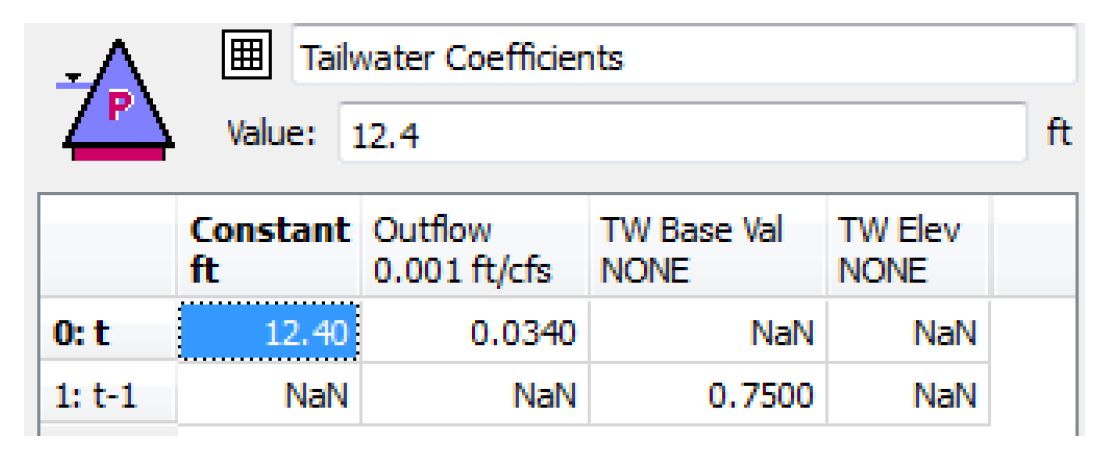Information: Not every cell will have a value, but there must be at least one value in the table. In addition, the coefficient for TW Elev at t cannot be non-zero or an error will be issued. (Tailwater Elevation at the current timestep is the value being computed, so it is not possible to use it in the calculation).
If any coefficients are specified (non-zero) for t-1 for Outflow, TW Base Val, or TW Elev, then the initial timestep value of those slots must also be specified.
I/O: Input only
Method Details
At the beginning of the run, the Tailwater Coefficients table is verified for valid coefficients as described in the slot information above. Not every coefficient has to be specified.
When the tailwater method is executed, the following computation is performed.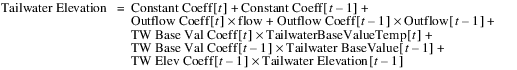Each coefficient and each series value are checked for validity before including in the total.
In the above equation, the values for flow and TailwaterBaseValueTemp are based on the information that is known:
• The flow variable represents the outflow passed into the method if valid, or the value on the Outflow slot if not passed in. If neither is valid, Tailwater Elevation is set to the previous Tailwater Elevation. If that is not valid, the method exits and waits for a flow value to become valid.
• The TailwaterBaseValueTemp is either the base value passed into the method or the value on the Tailwater Base Value slot. If neither is known, the previous Tailwater Base Value is used. If that is not valid, the method executes to wait for more information.Hoover Tailwater
The Hoover Tailwater method was developed by the USBR for use on Lake Mead. This method uses a table of empirically derived constants.
Slots Specific to This Method
Tailwater Base Value
Type: Series
Units: LENGTH
Description: base elevation of the tailwater
Information:
I/O: Optional; can be input or set by a link.
Tailwater Table
Type: Table
Units: FLOW vs LENGTH
Description: Reservoir Outflow vs either the Tailwater Elevation or the tailwater elevation increment
Information: If the Tailwater Base Value is non-zero, the Tailwater Table holds values of incremental increase in Tailwater Elevation over the Base value. Otherwise, the table holds the Tailwater Elevation values.
I/O: Required input
Hoover Tailwater Table
Type: Table
Units: NO UNITS
Description: constants for equation that calculates the Tailwater Elevation
Information: This method was developed by the USBR for use on Lake Mead. Column 1 is the TWaverage in feet, column 2 is hte5, column 3 is coeff1.
I/O: Required input
Method Details
The Hoover Tailwater user method performs calculations to determine the Tailwater Elevation. This method uses the following process.
1. The first step of this method is to set the temporary Tailwater Base Value (TWBaseValueTemp). TWBaseValueTemp is set equal to the Tailwater Base Value if the Tailwater Base Value was calculated by another user method or set via a link.
2. Then the previous timestep’s Tailwater Base Value is checked. If the previous timestep’s Tailwater Base Value is not known, a RiverWare error is posted and the run terminates.
The TWaverage, a local variable, is then calculated using the following process.
1. If TWBaseValueTemp is known: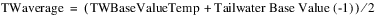2. If the temporary TWBaseValueTemp is NOT known, the TWaverage is set equal to the previous timestep’s Tailwater Base Value.
3. Once the TWaverage has been calculated, it is used to interpolate the empirical constants for the following equation from the Hoover Tailwater Table.
4. Finally,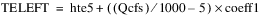5. Tailwater Elevation is then set equal to TELEFT (which is converted into internal units).
Related Terms
Following are definitions of some terms used in the these equations.
• TWBaseValueTemp—Temporary value for the Tailwater Base Value. This value may be determined from another method or propagated across a link.
• Tailwater Base Value (-1)—Tailwater Base Value at the previous timestep.
• TWaverage—Local variable that is used as the average of the Tailwater Elevation over the timestep.
• TELEFT—Tailwater Elevation in feet as determined by the equation given above.
• hte5—Empirical constant developed by the USBR.
• Qcfs—Flow, in cfs.
• coeff1—Empirical constant developed by the USBR.
Revised: 01/04/2021